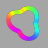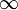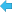Back to Adaptive Vision Studio website

You are here: Start » Filter Reference » Image Vector Transforms » ResizePixelVectors# ResizePixelVectors

Rescales an image treating pixels as vectors.

Name Type Range DescriptioninImage Image Input imageinRoi Region* Region of interestinVectorLength Real 0.0 -Desired vector length after normalizationoutImage Image Rescaled image

### Description

The filter treats pixels as a vector (each channel value as a coordinate). The operation scales pixels values to desired vector length:

\begin{aligned} \lvert \lvert Pixel \rvert \rvert &= \sqrt{Pixel.X^2 + Pixel.Y^2 + Pixel.Z^2 + Pixel.W^2}\\ Pixel.X &= \frac{Pixel.X}{\lvert \lvert Pixel \rvert \rvert}\cdot inVectorLength\\ Pixel.Y &= \frac{Pixel.Y}{\lvert \lvert Pixel \rvert \rvert}\cdot inVectorLength\\ Pixel.Z &= \frac{Pixel.Z}{\lvert \lvert Pixel \rvert \rvert}\cdot inVectorLength\\ Pixel.W &= \frac{Pixel.W}{\lvert \lvert Pixel \rvert \rvert}\cdot inVectorLength \end{aligned}

### Remarks

If a vector length of a pixel from an input image is zero, the output value equals zero.

If computed channel value exceeds its range, it is cut to maximal allowed value. Therefore the resulting vector length can be smaller than desired.

Computed values are rounded down to the next integer for integer pixel types.

### Hardware Acceleration

This operation supports automatic parallelization for multicore and multiprocessor systems.

### Complexity Level

This filter is available on Basic Complexity Level.

### See Also

• NormalizeImage – Rescales an image linearly, so that its minimum becomes inNewMinimum and the maximum of the remaining pixels becomes inNewMaximum.